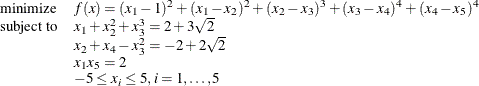### Example 8.5 Solving NLP Problems That Have Several Local Minima

Some NLP problems contain many local minima. By default, the NLP solver converges to a single local minimum. However, the NLP solver can search the feasible region for other local minima. After it completes the search, it returns the point where the objective function achieves its minimum value. (This point might not be a local minimum; see the SOLTYPE= option for more details.) Consider the following example, taken from Hock and Schittkowski (1981):The following statements call the NLP solver to search the feasible region for different local minima. The PERFORMANCE statement requests that the multistart algorithm use up to four threads. The SEED= option is specified for reproducibility, but it is not required in running the multistart algorithm.

proc optmodel;
var x{i in 1..5} >= -5 <= 5 init -2;

min f=(x - 1)^2 + (x - x)^2 + (x - x)^3 +
(x - x)^4 + (x - x)^4;

con g1: x + x^2 + x^3 =  2 + 3*sqrt(2);
con g2: x + x   - x^2 = -2 + 2*sqrt(2);
con g3: x*x = 2;

performance nthreads=4;
solve with nlp/multistart seed=1234 msmaxstarts=10;
print x.init x.msinit x;
quit;



The PRINT statement prints the initial point (x.init) that was specified in the INIT option of the VAR declaration; the starting point (x.msinit) that led to the best local solution; and finally the best local solution (x) that was found by the NLP solver in multistart mode. The SAS log is shown in Output 8.5.1.

Output 8.5.1: Progress of the Algorithm as Shown in the Log

 NOTE: Problem generation will use 4 threads. NOTE: The problem has 5 variables (0 free, 0 fixed). NOTE: The problem has 0 linear constraints (0 LE, 0 EQ, 0 GE, 0 range). NOTE: The problem has 3 nonlinear constraints (0 LE, 3 EQ, 0 GE, 0 range). NOTE: The OPTMODEL presolver removed 0 variables, 0 linear constraints, and 0 nonlinear constraints. NOTE: Using analytic derivatives for objective. NOTE: Using analytic derivatives for nonlinear constraints. NOTE: The NLP solver is called. NOTE: The Interior Point algorithm is used. NOTE: The MULTISTART option is enabled. NOTE: The deterministic parallel mode is enabled. NOTE: The Multistart algorithm is executing in single-machine mode. NOTE: The Multistart algorithm is using up to 4 threads. NOTE: Random number seed 1234 is used. Best       Local  Optimality    Infeasi-  Local  Local Start    Objective   Objective       Error      bility  Iters  Status 1   607.035801  607.035801  8.62585E-7  8.62585E-7      7  Optimal 2   607.035512  607.035512  2.81991E-7  5.88394E-9      7  Optimal 3   52.9025715  52.9025715  1.19566E-7  9.15988E-8      7  Optimal 4   52.9025715  52.9025734  4.37492E-7  6.32167E-8     13  Optimal 5 R 52.9025715  607.035793  5.31529E-7  4.33395E-7      8  Optimal 6   52.9025715  64.8740012  6.86447E-7  4.08169E-7      6  Optimal 7    27.871905   27.871905  7.19197E-7   1.4543E-7      5  Optimal 8    27.871905  27.8719052        5E-9   1.3246E-9      7  Optimal 9   0.02931102  0.02931102  5.51954E-7  5.42744E-7     10  Optimal 10   0.02931081  0.02931081        5E-7  2.66484E-7     11  Optimal NOTE: The Multistart algorithm generated 800 sample points. NOTE: 9 distinct local optima were found. NOTE: The best objective value found by local solver = 0.0293108081. NOTE: The solution found by local solver with objective = 0.0293108081 was returned.

The first column in the log indicates the index of the current starting point. An additional indicator (*, r, or R) can appear after the index to provide more information about the optimization run that started from the current point. For more information, see the section Iteration Log for Multistart. The second column records the best objective that has been found so far. Columns 3 to 6 report the objective value, optimality error, infeasibility, and number of iterations that the local solver returned when it was started from the current starting point. Finally, the last column records the status of the local solver—namely, whether it was able to converge to a local optimum from the current starting point.

The summaries and solution are shown in Output 8.5.2. Note that the best local solution was found by starting the local solver from a starting point (x.msinit) that is different from the point specified in the VAR declaration (x.init).

Output 8.5.2: Summaries and the Optimal Solution

The OPTMODEL Procedure

Problem Summary
Objective Sense Minimization
Objective Function f
Objective Type Nonlinear

Number of Variables 5
Bounded Above 0
Bounded Below 0
Bounded Below and Above 5
Free 0
Fixed 0

Number of Constraints 3
Linear LE (<=) 0
Linear EQ (=) 0
Linear GE (>=) 0
Linear Range 0
Nonlinear LE (<=) 0
Nonlinear EQ (=) 3
Nonlinear GE (>=) 0
Nonlinear Range 0

Performance Information
Execution Mode Single-Machine
Number of Threads 4

Solution Summary
Solver Multistart NLP
Algorithm Interior Point
Objective Function f
Solution Status Optimal
Objective Value 0.0293108146

Number of Starts 10
Number of Sample Points 800
Number of Distinct Optima 9
Random Seed Used 1234
Optimality Error 6.044933E-7
Infeasibility 3.8669805E-7

Presolve Time 0.00
Solution Time 3.67

 x.INIT x.MSINIT x
1 -2 -0.23856 1.1167
2 -2 2.93912 1.2205
3 -2 1.08486 1.5377
4 -2 -1.11094 1.9726
5 -2 1.80540 1.7910

Alternatively, the following SAS statements show how you can add the NODES= option in the PERFORMANCE statement to run this example in distributed mode.

Note: SAS High-Performance Optimization software must be installed before you can invoke the MULTISTART option in distributed mode.

proc optmodel;
var x{i in 1..5} >= -5 <= 5 init -2;

min f=(x - 1)^2 + (x - x)^2 + (x - x)^3 +
(x - x)^4 + (x - x)^4;

con g1: x + x^2 + x^3 =  2 + 3*sqrt(2);
con g2: x + x   - x^2 = -2 + 2*sqrt(2);
con g3: x*x = 2;

performance nodes=4 nthreads=4;
solve with nlp/multistart seed=1234 msmaxstarts=10;
print x;
quit;



The SAS log is displayed in Output 8.5.3.

Output 8.5.3: Progress of the Algorithm as Shown in the Log

 NOTE: Problem generation will use 4 threads. NOTE: The problem has 5 variables (0 free, 0 fixed). NOTE: The problem has 0 linear constraints (0 LE, 0 EQ, 0 GE, 0 range). NOTE: The problem has 3 nonlinear constraints (0 LE, 3 EQ, 0 GE, 0 range). NOTE: The OPTMODEL presolver removed 0 variables, 0 linear constraints, and 0 nonlinear constraints. NOTE: Using analytic derivatives for objective. NOTE: Using analytic derivatives for nonlinear constraints. NOTE: The NLP solver is called. NOTE: The Interior Point algorithm is used. NOTE: The MULTISTART option is enabled. NOTE: The deterministic parallel mode is enabled. NOTE: The Multistart algorithm is executing in the distributed computing environment with 4 worker nodes. NOTE: The Multistart algorithm is using up to 4 threads. NOTE: Random number seed 1234 is used. Best       Local  Optimality    Infeasi-  Local  Local Start    Objective   Objective       Error      bility  Iters  Status 1   607.035871  607.035871  8.43836E-7  8.43836E-7      8  Optimal 2   52.9025792  52.9025792  2.39856E-7  2.84012E-8      7  Optimal 3   52.9025792  607.035521  4.17297E-7  4.17297E-7      8  Optimal 4   52.9025792  52.9025794  6.69221E-8  3.26907E-9      8  Optimal 5   52.9025792  64.8739968   2.9959E-7  1.19421E-7      8  Optimal 6   52.9025792  52.9026071  3.70891E-7  3.70891E-7     11  Optimal 7   0.02931083  0.02931083  2.53214E-7  3.54144E-9     10  Optimal 8   0.02931083  52.9025015  9.03254E-7  9.03254E-7      5  Optimal 9   0.02931083  52.9025916  7.08702E-7  7.08702E-7      6  Optimal 10   0.02931083  52.9026134  4.08103E-7  4.08103E-7      8  Optimal NOTE: The Multistart algorithm generated 1600 sample points. NOTE: 10 distinct local optima were found. NOTE: The best objective value found by local solver = 0.0293108314. NOTE: The solution found by local solver with objective = 0.0293108314 was returned.

Output 8.5.4 shows the summaries and solution. Note that the Performance Information table shows that four computing nodes with four threads on each node are used in distributed mode.

Output 8.5.4: Summaries and the Optimal Solution

The OPTMODEL Procedure

Problem Summary
Objective Sense Minimization
Objective Function f
Objective Type Nonlinear

Number of Variables 5
Bounded Above 0
Bounded Below 0
Bounded Below and Above 5
Free 0
Fixed 0

Number of Constraints 3
Linear LE (<=) 0
Linear EQ (=) 0
Linear GE (>=) 0
Linear Range 0
Nonlinear LE (<=) 0
Nonlinear EQ (=) 3
Nonlinear GE (>=) 0
Nonlinear Range 0

Performance Information
Host Node << your grid host >>
Execution Mode Distributed
Grid Mode Symmetric
Number of Compute Nodes 4
Number of Threads per Node 4

Solution Summary
Solver Multistart NLP
Algorithm Interior Point
Objective Function f
Solution Status Optimal
Objective Value 0.0293108314

Number of Starts 10
Number of Sample Points 1600
Number of Distinct Optima 10
Random Seed Used 1234
Optimality Error 2.5321436E-7
Infeasibility 3.5414378E-9

Presolve Time 0.00
Solution Time 3.99

 x
1 1.1167
2 1.2204
3 1.5378
4 1.9727
5 1.7911